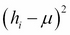•#### Learning Bayesian Models with R#### Overview of this book

Learning Bayesian Models with RCreditswww.PacktPub.comPrefaceFree Chapter
Introducing the Probability TheoryThe R EnvironmentIntroducing Bayesian InferenceMachine Learning Using Bayesian InferenceBayesian Regression ModelsBayesian Classification ModelsBayesian Models for Unsupervised LearningBayesian Neural NetworksBayesian Modeling at Big Data ScaleIndex## Exercises

1. Derive the equation for the posterior mean by expanding the square in the exponentialfor each i, collecting all similar power terms, and making a perfect square again. Note that the product of exponentials can be written as the exponential of a sum of terms.
2. For this exercise, we use the dataset corresponding to Smartphone-Based Recognition of Human Activities and Postural Transitions, from the UCI Machine Learning repository (https://archive.ics.uci.edu/ml/datasets/Smartphone-Based+Recognition+of+Human+Activities+and+Postural+Transitions). It contains values of acceleration taken from an accelerometer on a smartphone. The original dataset contains x, y, and z components of the acceleration and the corresponding timestamp values. For this exercise, we have used only the two horizontal components of the acceleration x and y. In this exercise, let's assume that the acceleration follows a normal distribution. Let's also assume a normal prior distribution for the mean values of...